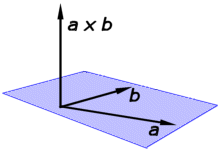Go to contentThe vector (or cross) product of the vectors a and b is defined to be:
a \times b = |a| |b| \sin \theta \hat{n}
where \theta is the angle between the vectors and \hat{n} is a unit vector perpendicular to both a and b.The direction of \hat{n} is given by the right-hand rule - see the diagram.

The magnitude the cross product is equal to the area of the parallelogram that the vectors span.

If i, j and k are the familiar unit vectors, then:
i \times j = k, j \times k = i, k \times i = j and
i \times i = 0, j \times j = 0, k \times k = 0

If a = a_1i+a_2j+a_3k \quad and b = b_1i+b_2k+b_3k \quad then: a \times b = (a_2b_3-a_3b_2)i + (a_3b_1-a_1b_3)j + (a_1b_2-a_2b_1)kYou can get a better display of the maths by downloading special TeX fonts from jsMath. In the meantime, we will do the best we can with the fonts you have, but it may not be pretty and some equations may not be rendered correctly.

## Glossary

### cross product

For vectors a and b, the cross product is the vector c whose magnitude is ab sin C, where C is the angle between the directions of the vectors, and which is perpendicular to both a and b.

### magnitude

A measure of the size of a mathematical object

### perpendicular

one line being at right angles to another.

### rule

A method for connecting one value with another.

### union

The union of two sets A and B is the set containing all the elements of A and B.

### unit vector

A vector with magnitude equal to 1.

### vector

A mathematical object with magnitude and direction.

Full Glossary List

## This question appears in the following syllabi:

SyllabusModuleSectionTopicExam Year
AQA A-Level (UK - Pre-2017)FP4VectorsCross product-
AQA A2 Further Maths 2017Pure MathsVectorsCross Product-
AQA AS/A2 Further Maths 2017Pure MathsVectorsCross Product-
CBSE XII (India)Vectors and 3-D GeometryVectorsCross product definition, geometrical interpretation, properties and application-
CCEA A-Level (NI)FP3VectorsCross product-
Edexcel A-Level (UK - Pre-2017)FP3VectorsCross product-
Edexcel AS Further Maths 2017Further Pure 1VectorsCross Product-
Edexcel AS/A2 Further Maths 2017Further Pure 1VectorsCross Product-
I.B. Higher Level4VectorsCross product-
Methods (UK)M4VectorsCross product-
OCR A-Level (UK - Pre-2017)FP3VectorsCross product-
OCR AS Further Maths 2017Pure CoreVectorsCross Product-
OCR MEI A2 Further Maths 2017Core Pure BVectors and 3-D SpaceCross Product-
OCR-MEI A-Level (UK - Pre-2017)FP3VectorsCross product-
Pre-Calculus (US)E1VectorsCross product-